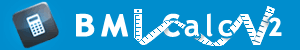# Body Mass Index Formula

BMI formula is the result of investigations performed by Belgian scientist Adolphe Quetelet. Although BMI formula was discovered in the 19th century, it became really popular in the second part of the 20th century. Particular interest in BMI formula is explained by the fact that wide spread obesity became a characteristic feature of economically developed countries.
BMI formula allows calculating BMI - body mass index. BMI formula describes body condition taking into account weight and height of the person. At the same time BMI formula does not measure directly the amount of body fat. According to BMI formula body weight is divided by the square of height. For a height given, BMI is proportional to mass, but for a mass given, BMI is inversely proportional to squared height. Doctors usually express BMI formula in kg/m2. However, BMI formula can be used for English Units.

## BMI formula - English Units

BMI =mass (lb) * 703
(height (in))2

Where lb = pound; in = inch.

## BMI formula - Metric Units

BMI =mass (kg)
(height (m))2

Where kg = kilogram; m = meter.

BMI formula is regarded as a useful method of classification for individuals with different body mass. BMI formula is an accessible mathematical measure of thinness, norm, and fatness. BMI formula helps doctors explain with clear and understandable language such notions as over-weight and under-weight.

### Formula for BMI calculator - English Units

BMI = ( Weight in Pounds / ( Height in inches x Height in inches ) ) x 703

### Formula for BMI calculator - Metric Units

BMI = ( Weight in Kilograms / ( Height in Meters x Height in Meters ) )